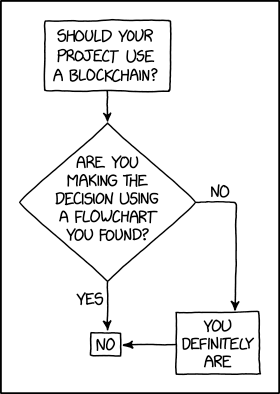# How to Convert Epoch Time to Date Time in Python

## Problem Formulation and Solution Overview

In this article, you’ll learn how to convert Epoch Time to a Date Time representation using Python.

On January 1st, 1970, Epoch Time, aka Time 0 for UNIX systems, started as a date in history to remember. This date is relevant, not only due to this event but because it redefined how dates are calculated!

To make it more fun, we will calculate the time elapsed in Epoch Time from its inception on January 1, 1970, to January 1, 1985, when the first mobile phone call was made in Britain by Ernie Wise to Vodafone. This will then be converted to a Date Time representation.

💬 Question: How would we write code to convert an Epoch Date to a Date Time representation?

We can accomplish this task by one of the following options:

## Method 1: Use fromtimestamp()

This method imports the `datetime` library and calls the associated `datetime.fromtimestamp()` function to convert Epoch Time into a Local Date Time representation.

To run this code error-free, install the required library. Click here for installation instructions.

```import datetime

epoch_time = 473398200
date_conv = datetime.datetime.fromtimestamp(epoch_time)
print(date_conv.strftime('%d-%m-%Y'))```

Above, imports the `datetime` library. This allows the conversion of an Epoch Time integer to a readable Local Date Time format.

The following line declares an Epoch Time integer and saves it to `epoch_time`.

Next, the highlighted line converts the Epoch Time into a Local Date Time representation and saves it to `date_conv`. If output to the terminal at this point, it would display as follows:

Finally, `date_conv `converts into a string using `strftime()` and outputs the formatted date to the terminal.

## Method 2: Use time.localtime()

This method imports the `time` library and calls the associated `time.localtime()` function to convert Epoch Time into a Local Date Time representation.

```import time

epoch_time = 473398200
date_conv = time.localtime(epoch_time)
print(date_conv)```

Above, imports the `time `library. This allows the conversion of an Epoch Time to a readable Local Date Time format.

The following line declares an Epoch Time integer and saves it to `epoch_time`.

Next, the highlighted line converts the Epoch Time into a Local Date Time representation and saves it to `date_conv` as a Tuple as shown below:

The appropriate elements will need to be accessed to format a date or time. For this example, we will construct the date.

`print(f'0{date_conv}-0{date_conv}-{date_conv}')`

The output is as follows:

## Method 3: Use datetime.utcfromtimestamp

This method imports the `datetime `library and calls the associated `datetime.utcfromtimestamp()` function to convert an Epoch Time into a UTC Date Time representation.

To run this code error-free, install the required library. Click here for installation instructions.

```import datetime

epoch_time = 473398200
date_conv = datetime.datetime.utcfromtimestamp(epoch_time).strftime('%Y-%m-%d %H:%M:%S')
print(date_conv)```

Above, imports the `datetime` library. This allows the conversion of an Epoch Time integer to a readable UTC Date Time format.

The following line declares an Epoch Time integer and saves it to `epoch_time`.

Next, the highlighted line accomplishes the following:

The output is sent to the terminal.

💡Note: Universal Time (UTC) is the primary standard 24-hour time clock by which the World regulates clocks and time.

## Method 4: Use time.localtime() and time.strftime()

This method imports the `time` library in conjunction with the `time.localtime()`and `time.strftime()` functions to convert Epoch Time into a Local Date Time representation.

```import time

epoch_time = 473398200
date_conv = time.strftime('%c', time.localtime(epoch_time))
print('Formatted Date:', date_conv)```

Above, imports the `time `library. This allows the conversion of an Epoch Time to a readable Local Date Time format.

The following line declares an Epoch Time integer and saves it to `epoch_time`.

Next, the highlighted line converts the Epoch Time into a Local Date Time representation, converts to a string (`strftime()`) format and saves it to `date_conv`.

The output is sent to the terminal.

## Summary

These four (4) methods of converting an Epoch Time to a Date Time representation should give you enough information to select the best one for your coding requirements.

Good Luck & Happy Coding!

## Programmer Humor – Blockchain“Blockchains are like grappling hooks, in that it’s extremely cool when you encounter a problem for which they’re the right solution, but it happens way too rarely in real life.” source – xkcd